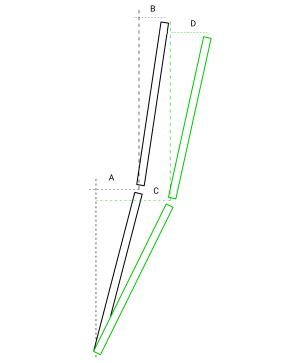Measurand's SAAView application provides various deviation and displacement graphs for visualizing ShapeArray™ data collected over a period of time. To fully understand the different deviation and displacement visualizations, you must understand the idea of deviation with respect to the ShapeArray™ instrument.

Simply put, in vertical installations, deviation is the distance a segment's vertex is from perpendicular (relative to gravity). Displacement is the difference between a vertex's deviation in a reading and its deviation in a baseline reading. The following figure presents a very simplified diagram to help illustrate this.Figure 1: A two segment ShapeArray™ instrument in two separate positions

In this diagram, there is a two segment ShapeArray™ instrument in two separate positions. The black segments represent position 1 and the green segments represent position 2. The deviation of the bottom segment in the first reading is the distance represented by "A" and the deviation of the top segment in the first reading is the distance represented by "B".

With this foundation, we can more easily explain the different deviation and displacement graphs in SAAView. Let's start with the different deviation graphs.

• Incremental Deviation - This is simply the deviation of each segment's vertices plotted on the graph. For the first reading, this means the distances labeled "A" and "B" will be plotted for the first reading and "C" and "D" will be plotted for the second reading.
• Cumulative Deviation (Absolute Shape) - The deviation of each segment's vertices from the reference point are plotted on the graph. This is effectively the sum of all vertices' deviations from the reference point plotted at each elevation. Using the simple example in the figure above, for the first reading, we would plot "A" for the bottom segment, and "A + B" for the top segment. For the second reading, we would plot "C" for the bottom segment and "C + D" for the top segment.

With an understanding of deviation, we can examine the various displacement graphs. Displacement is defined as the change in deviation between a reading and the baseline reading. It is calculated by subtracting the baseline reading's deviation from the current sample's deviation. This difference is the amount of movement that occurred between the readings. For example, the displacement of the bottom segment's top vertex would be the difference between "C" and "A".

• Incremental Displacement - The displacement for each segments' vertices is plotted on the graph. Using our simplified example in the figure above, the displacement of the first reading would be zero at all elevations. The displacement for the second reading would be "C - A" for the bottom segment and  "D - B" for the top segment.
• Cumulative Displacement (Deformation) - Cumulative displacement is the sum of all displacements of all segments from the zero position at each elevation. The cumulative displacement for the first reading at all elevations would be zero. The cumulative displacement for the second reading would be "C - A" for the bottom segment and "(C - A) + (D - B)" for the top segment.